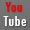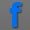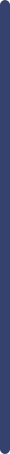fundamentals of interface chemistry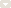measure method of surface tensiometermeasure method of contact angle metermeasure method of spinning drop tensiometerReferences and ApplicationsDictionary and GlossaryGlossary A-GGlossary H-MGlossary O-TGlossary U-ZStandardsVideo Collection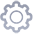product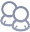about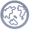NewsView Products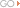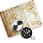contact us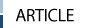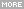> HOME > fundamentals of interface chemistry >Contact angle on rough or chemically heterogeneous surface--Theory of surface tension, contact angle, wetting and work of adhesion (3)
 Contact angle on rough or chemically heterogeneous surface--Theory of surface tension, contact angle, wetting and work of adhesion (3)1.3 Contact angle on rough or chemically heterogeneous surface Real surfaces are usually rough or chemically heterogeneous, of both. On rough surfaces, the local angle of inclination of the solid surface with respect to some reference plane varies from point to point. Similarly, on chemically heterogeneous surfaces, the chemical composition is different at various locations. Therefore, two fundamental questions are: What is the value of the actual CA (Fig. 1b)? and what is the experimentally measurable quantity? The advancing and receding contact angles can be relatively well defined from an experimental point of view. The hysteresis range can be currently used in only two ways: (a) as an empirical measurement of the extent of heterogeneity of solid suface; and (b) as a means to estimate the most stable CA, CA ms Two suggestion for the latter option have been given in the literature. One is       COSθ ms  = (COSθa + COSθr)/2                                                (1.6) And the other is               θ ms  = (θa + θr)/2                                                            (1.7) And a preferred approach is Chibwski  method for calculating surface free energy, as described in next section of surface free energy. The most stable CA is experimentally less accessible than the advancing or receding CA; however, its theory is well defined. Some years ago it was proven that the equations developed intuitively by Wenzel, Cassie and Baster are good approximations for the most stable CA if the drop size is sufficiently large compared with the scale of roughness or heterogeneity. For a rough but chemically homogeneous solid surface, the surface area is larger than its projected area by a factor r , which is called the roughness ratio. Now, each term contributing to the Gibbs energy of a wetting system consists of a product of the corresponding interfacial tension by the interfacial area, therefore, the increase in the solid-liquid and solid-fluid interfacial areas by a factor of r can be mathematically viewed as an increase in the interfacial tensions by the same factor. This immediately leads to the Wenzel equation: COSθ W  = r COSθY                                                          (1.8)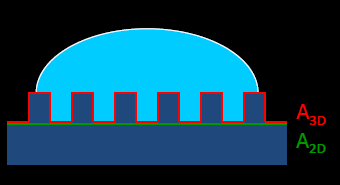Where the subscript W indicates the apparent CA calculated according to this equation. Initial experiments seem to confirm the theoretical validity of these equations for sufficiently large drops. For a smooth, but chemically heterogeneous surface, the same approach of averaging the interfacial tensions immediately leads to the Cassie equation  for the apparent CA, θ C COSθ C  =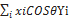(1.9a)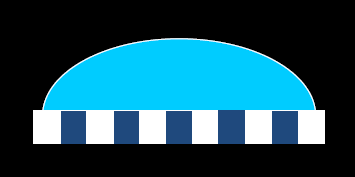In this equation, xi is the area fraction characterized by a given chemistry i. This is a generalized form of the original Cassie equation that referred to only two chemistries. The summation can be replaced by an integral when the chemical heterogeneity is continuously varying over the surface. Similarly to the rough surface case, two-dimensional and three-dimensional numerical simulations showed that the Cassie CA becomes a very good approximation for the most stable CA as the drop becomes larger in comparison with the scale of heterogeneity. A rough surface may, of course, also be chemically heterogeneous. A special case of roughness and chemical heterogeneity occurs when air is trapped under the drop within the roughness grooves. This phenomenon is of great interest and importance, especially with regard to super-hydrophobic surface. The approximate equation for the most stable CA in this case is the Cassie-Baxter equation , which is actually a combination of the Wenzel and Cassie equation. This equation is of fundamental importance for designing super-hydrophobic surface, but somewhat of less interest for the assessment of γSv : COSθ W  =f Ls COSθY - f LV                                               (1.9b)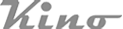Copy Right(C)2005-2014 USA KINO Industry Co., LTD. TEL:+1(212) 6555-777 sales@uskino.com. All rights reserved. Sitemap Follow US: